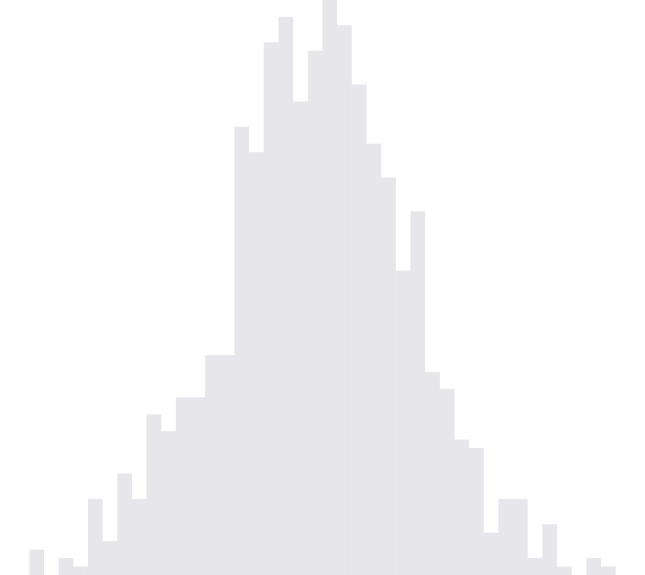Info, Uncertainty

27 Jun 2013

# Problem from Random Iterated Functions

Here’s a simple problem discussed by Persi Diaconis and David Freedman which motivates the beautiful theory of random iterated functions, a way of thinking about Markov chains that unifies diverse ideas from math, physics, and statistics.

The particular problem is to estimate the stationary distribution of the Markov chain $X_{n}$ defined on $[0, 1]$ by the following procedure: Let $X_{n + 1}$ be uniformly distributed on the interval to the left of $x_{n}$ with probability $1/2$, else draw $X_{n + 1}$ uniformly from the interval to the right of $x_{n}$.

More formally, $X_{n}$ is a Markov chain with the transition kernel

This chain is irreducible and aperiodic, so converges to a unique stationary distribution $\pi$. To find it, recall that $\pi$ is a stationary distribution for a chain with kernel $K$ if and only if $\pi K = \pi$. Hence, $\pi$ must satisfy

Applying the fundamental theorem of calculus, we find that the stationary distribution $\pi$ is the solution to the ODE

To solve this ODE, we integrate

Hence, we find that $\pi$ must be the arcsine distribution.

This result might be a little surprising: we start with a scheme that uniformly choose between two uniform distributions, and end up with a stationary distribution that is far from uniform. However, consider that, if $X_{n}$ is already close to one of the edges, then $X_{n + 1}$ has a 50% chance of being even closer to that edge.

Below, we have include code for taking samples from $X_{n}$. Further, we illustrate the convergence of samples from an instance of the $X_{n}$. We notice that the histogram begins to take on the characteristic heavy-tailed shape of the arcsine distribution as the sample size becomes larger and larger.